``` 1. 45316 + 52131 – 65229 =? + 15151

(a) 17063

(b) 17073

(c) 17076

(d) 17067

(e) None of these

2. 27% of 510 + ? = 266.3

(a) 182.6

(b) 122.6

(c) 123.6

(d) 128.6

(e) None of these

3.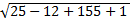=?

(a) 13

(b) 14

(c) 17

(d) 16

(e) None of these

4. (23 × 23 × 23 × 23 × 23 × 23)5 × (23 × 23)2 ÷ (23)2 = (23)?

(a) 32

(b) 30

(c) 9

(d) 7

(e) 11

5.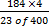=?

(a) 7

(b) 9

(c) 8

(d) 5

(e) None of these

6. (62.5 × 14 × 5) ÷ 25 + 41 = (?)3

(a) 4

(b) 5

(c) 9

(d) 8

(e) 6

7. What will come in place of both the question marks (?) in the following question?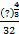=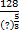(a) 16

(b) 12

(c) 18

(d) 14

(e) None of these

8. (41+7×9)÷7+(25.153)=?

(a) 40

(b) 23

(c) 25

(d) 35

(e) None of these

9. If (11)3 is subtracted from (46)2 what will be the remainder?

(a) 787

(b) 785

(c) 781

(d) 783

(e) None of these

10. 4 × 566 ÷ 5 + 24.2 – 36 = (?)2

(a) 20

(b) 21

(c) 22

(d) 23

(e) 25

11. The sum of the squares of two odd numbers is 11570.
The square of the smaller number is 5329.
What is the other number?

(a) 73

(b) 75

(c) 78

(d) 79

(e) None of these

12.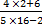=?

(a) 5

(b) 16/35

(c) 1/5

(d) 16/39

(e) None of these

13. If the square of a number is subtracted from 4052 and the difference is multiplied by 15,
the answer so obtained is 41340. What is the number?

(a) 36

(b) 1024

(c) 32

(d) 1296

(e) None of these

14. 7480 × 2/3 × 3/4 × 5/8 =?

(a) 2330

(b) 2337.8

(c) 2530

(d) 2373.5

(e) None of these

15. 12/13 + 1/26 + 1 1/13 =?

(a) 1 1/26

(b) 2 1/26

(c) 1 3/26

(d) 11/26

(e) None of these

16. 4/5th of 38% of 600 – 15.4 =?

(a) 169

(b) 163

(c) 165

(d) 168

(e) None of these

17. 5/7 + 2/3 - 2/7 =?

(a) 1 1/21

(b) 1 2/21

(c) 2 1/21

(d) 2 2/21

(e) None of these

18. 42% of 12% of 1/4th of 15000 =?

(a) 188

(b) 182

(c) 185

(d) 187

(e) None of these

19. 56835 – 12683 + 38934 =?

(a) 83085

(b) 83083

(c) 83088

(d) 83086

(e) None of these

20. 12% of 150 + 62% of 800 =?

(a) 516

(b) 518

(c) 515

(d) 514

(e) None of these